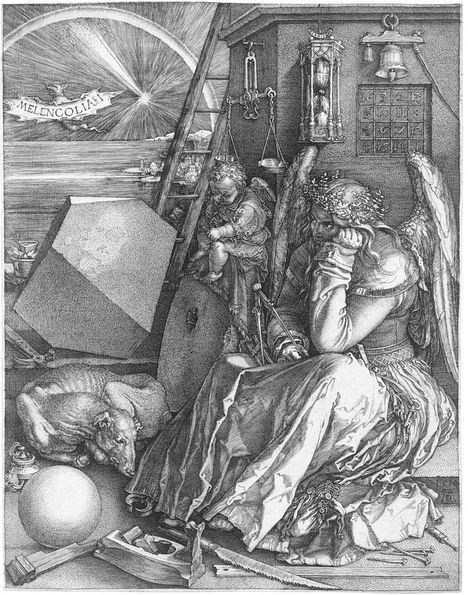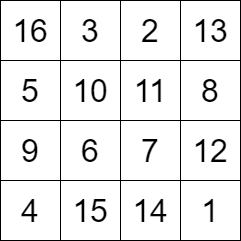# Melencolia IishtupeedCriterion 2021 Round 12
Limits 1s, 512 MBAccording to Wikipedia, Albrecht Dürer (May 21, 1471 - April 6, 1528) was a German painter, printmaker, and theorist of the German Renaissance. His famous engraving “Melencolia I”, as shown in the figure above, contains references to carpentry, alchemy, geometry, numerology, and many other disciplines. It has been the subject of more scholarship than probably any other print. In the engraving, a square with an interesting property can be seen. The square is shown below:The sum of the values in each row and each column is equal to 34. Even the sums of the values in the main diagonal and the anti-diagonal are 34. This type of square is also known as Magic Square.

In this problem, your task is to find out the number of ways to fill a $4\times 4$ grid to make Magic Squares. Two Magic Squares are considered different if they differ by at least one cell. To keep the problem simple, you will be given an integer $N$. You need to construct the Magic Square using integers in the range $[0, N]$. You can use any integer as many times as you want.

## Input

The input will contain a single integer, $N (0 \leq N \leq 10)$ denoting the range.

## Output

Print one integer denoting the possible number of different Magic Squares.

## Sample

InputOutput
1

34


### Submit